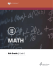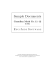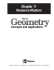# MP Board 10th Sample paper for Mathematics

## Transcription

MP Board 10th Sample paper for Mathematics
```MP Board 10th Sample paper for Mathematics
General Instructions:
1. All questions are compulsory.
2. The question paper consists of 29 questions divided into three sections-A, Section-B, Section-C.



Section A comprises of 10 questions of one mark each,
section B comprises of 12 questions of 4 marks each
section C comprises of 7 questions of six marks each.
3. All questions in Section A are to be answered in one word, one sentence or as per the
exact requirement of the question.
4. Marks are allotted to each question for your convenience.
SECTION A (One Mark Questions)
Question-1: If the equation kx2 + 4x+ k=0 has two equal roots, then
(a) k = ± 2
(b) k = 0
(c) k = 2
(d) none of these
Question-2: If the sum of n terms of an A.P., is 2n2 +4n, then its nth term is
(a) 4n + 2
(b) 4n + 5
(c) 10n - 4
(d) none of these
Question-3: If tangents TA and TB from a point T to a circle with center O are inclined to each other
at an angle of 70° then ∠TOA is equal to
(a) 80°
(b) 70°
(c) 55°
(d) 50°
Question-4: To divide a line segment AB in the ratio 5:3, first a ray AX is drawn so that BAX is an
acute angle and then at equal distances points are marked on the ray AX such that the minimum
number of these points is
(a) 12
(b) 11
(c) 10
(d) 8
Question-5: If the circumference and area of a circle are numerically equal,then diameter of the
circle is
(a) 2x
(b) 2
(c) 4
(d) 4x
Question-6: The number of solid spheres, each of diameter 2 cm that could be moulded to form a
solid metal cylinder of height 45 cm and diameter 4 cm is
(a) 14
(b) 15
(c) 135
(d) none of these
Question-7: From the top of a cliff 20 m high the angle of elevation of a tower is found to be equal to
the angle of depression of the foot of the tower. The height of the tower is
(a) 40 m
(b) 60 m
(c) 90 m
(d) none of these
Question-8: If the distance between the points (5,r) and (1,0) is 5,then r is
(a) 5
(b) -5
(c) 0
(d) ±3
Question-9: The area of a triangle with vertices A(4,0), B(7,0) and C(9,5) is
(a)14 sq. units
(b) 28 sq. units
(c) 17.5 sq. units
(d) none
Question-10: If A(5,-1),B(-3,-2) and C(-1,8) are the vertices of triangle ABC, then the length of the
median through A is
(a)√50 units
(b)√60 units
(c)√62 units
(d)√65 units
SECTION B(Four Mark Questions)
Question-11: Does there exist a equation whose coefficients are rational but both of its roots are
Question-12: The nth term of an A.P.,cannot be n2+1.Justify your answer.
Question-13: AB is a chord of the circle and ∠AOC is its diameter such that ∠ACB = 50°. If AT is the
tangent to the circle at the point A, then BAT is equal to 50°. Justify your answer.
Question-14: Write True or False and give reason for your answer for the following:A pair of
tangents can be constructed from a point P to a circle of radius 6 cm situated at a distance of 5 cm
from the centre.
Question-15: Is it true that the distance traveled by a circle wheel of diameter x cm in one revolution
is 2πx cm? Why?
Question-16: A circle is inscribe d in a square of side x cm and another circle is circumscribing the
square. Is it true to say that area of the outer circle is two times the area of the inner circle? Give
Question-17: Write True or False and justify you answer for the following:
A spherical steel ball is melted to make 10 new identical balls. Then, the radius of each new ball is
1/10th radius of the original ball.
Question-18: A hemisphere is cut out from the top of the cylinder with radius equal to the radius of
cylinder. Taking radius as r and height of cylinder as h. find total surface area of solid ?
SECTION C(Six mark Questions)
Question-19: Solve the following quadratic equation: 3x2+2x-3
Question-20: A bag contains cards which are numbered from 2 to 100. A card is drawn at random
from the bag. Find the probability that it bears
(i) a two digit number
(ii) a number which is a perfect square
Question-21: In an AP,the sum of first ten terms is -150 and the sum of its next ten terms is -550.
Find the AP.
Question-22: Construct a triangle ABC in which BC=9 cm,∠B=60° and AB=6 cm,then construct
another triangle whose sides are
2/3 of the corresponding sides of ∠ABC.
Question-23: If (1,2),(4,y),(x,6) and (3,5) are the vertices of a parallelogram taken in order, find the
values of x and y.
Question-24: Two tangents PA and PB are drawn to a circle with center O from an external point P.
prove that ∠APB=2 ∠OAB.
Question-25: A pole 5 m high is fixed on the top of a tower.The angle of elevation of the top of the
pole as observed from a point A on the ground is 60° and angle of depression of point A from the top
of the tower is 45°.Find the height of the tower. [Take 3 =1.73].
Question-26:A coin is tossed 3 times. List the possible outcomes. Find the probability of getting at
Question-27: ABC is a triangle right angled at A.Semicircles are drawn on AB,AC and BC as
diameters. Find the area of the shaded region.
Question-28: A canal is 300 cm wide and 120 cm deep.The water in the canal is following with a
speed of 20 km/h.How much area will it irrigate in 20 minutes if 8 cm of standing water is desired?
```

### square, circle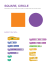### CBSE Sample Papers 2015 : X Mathematics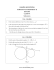### Maths Sample Papers for Class 10 CBSE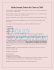### Name: __________________________________________________________________ Period: ___________________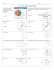### Sample Paper 2014 Class – X Subject –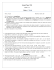### Mathematics - Paper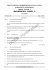### fire stone home products outback chair company laneventure gloster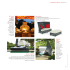### Final Review Finally - Mr. Mayer AfterMath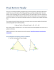### SAMPLE QUESTION PAPER - I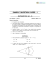### Graphical Methods for Describing Data 6` 57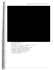### January.2013 - Fitness oprema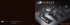### mega-bergerac ® circle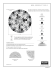### Circle Geometry Practice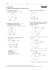### Jarus, T., Barnea, R., Wasserlauf, N., Boretz, L., Yakoel, S., Galon, I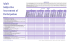### CLASS X CIRCLES 1. Two tangents PA and PB are drawn to the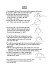### book here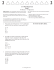### Pi Day: Making Cylinders â Teacher Material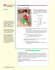### CH 41 - CIRCLES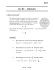### 6th Grade | Unit 6